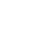# Filter Events Based on a Predicate with RxJS filter

Published 6 years ago
Updated 8 months ago

through a predicate function that tells what is the criteria to pass or reject an event. This lesson introduces filter: an operator that allows us to let only certain events pass, while ignoring others.

🚨 Since we are importing `interval` from RxJS, we don't need to preface our Observables with `Rx.Observable`. You can no longer `.{operator}`, you need to `.pipe({operator})` instead.

[00:00] The next operator we're going to see is Filter. Suppose we have an observable of numbers, like zero, one, two, three, etc., which is generated by that interval function above, and we would like to have only even numbers. We want to ignore out the odd numbers like one, three, five, seven, etc.

[00:22] We do that with Filter. It allows us to remove some of events by giving a function which is called a predicate. Predicate functions are simply they take an argument and they should return true or false. That's what a predicate function means.

[00:37] We return true when this X, which is the input event on this input observables, should pass. If it passes, it will appear here on the bottom. We return false when it should be ignored. In case of odd and even numbers, if the modulo of X with two is zero, then that's an even number, and this comparison would be true. That's why even numbers would pass.

[01:04] But if this modulo two with X would be one, then that means that we have an odd number, and that's why this comparison would be false, and the event would not pass. One does not pass, for instance. It would return false.

[01:20] Two would pass so the predicate would return true, and then etc., and we get an observable output at the bottom here with only even numbers. Let's call that in actual JavaScript. We take the modulo with two, check if it's zero, and then this is now observable bar.

[01:43] If we run this, we see that bar has only even numbers. Foo is ticking every second, but every other second it gives an odd number which we ignore. To recap, Filter allows you to pick certain events, and ignore others, through a predicate function that tells what is the criteria to pass or reject an event.Erkan Buelbuel
~ 5 years ago

Why don't you use iterable JSON structures in your demos? Nobody would use "Observables" for primitive arrays.André Staltzinstructor
~ 5 years ago

Hi EBIA,

Which arrays are you referring to in this lesson?Daniel Laubacher
~ 2 years ago

I would like to note that this isn't Rx 6 friendly. You'll need to use these concepts with slightly different imports and usage.